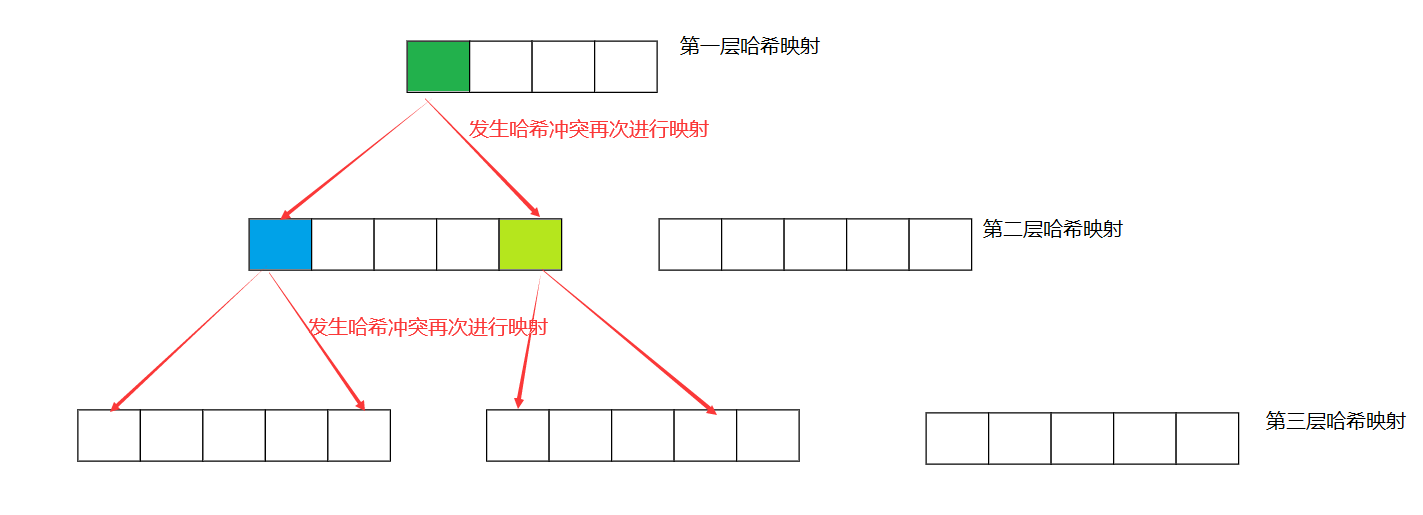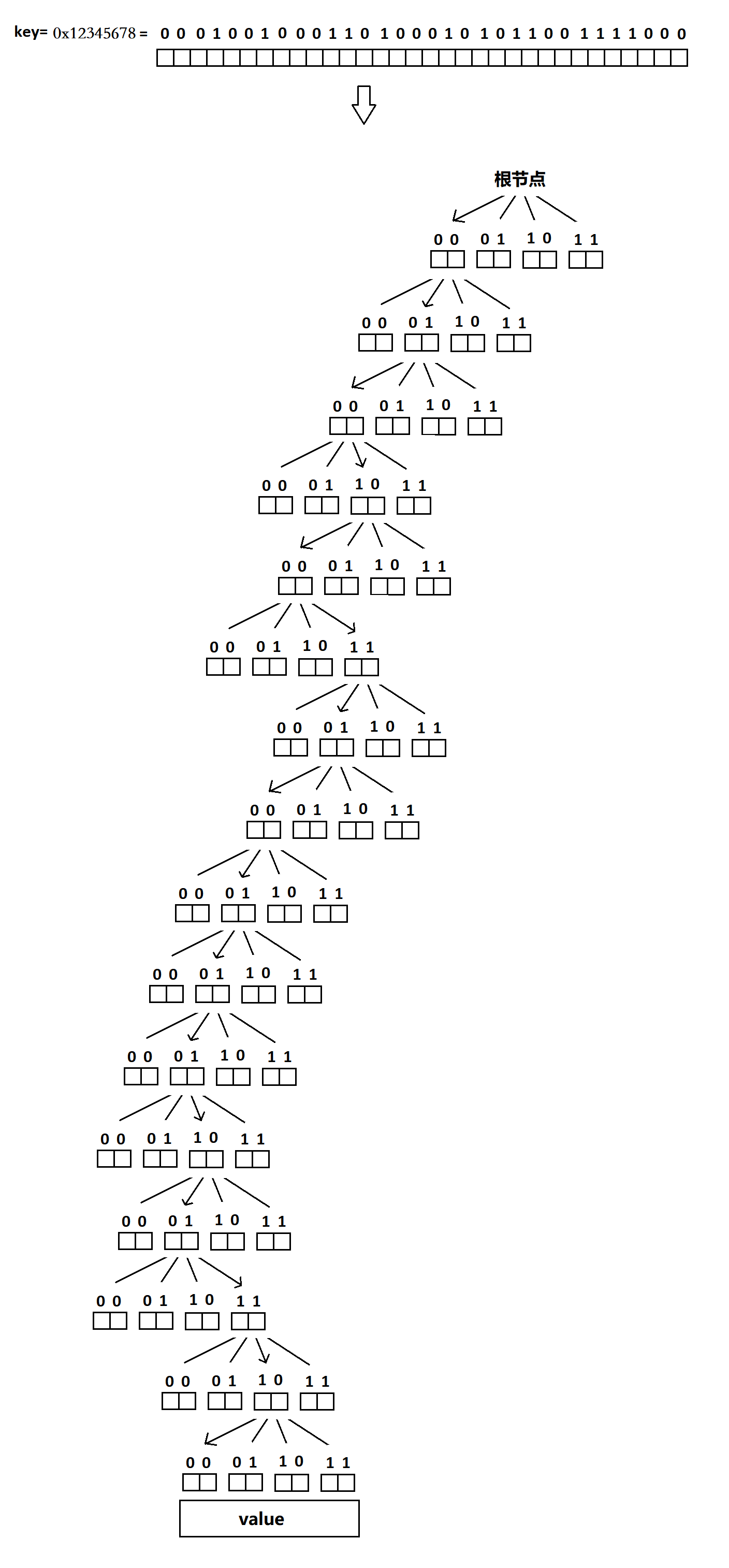• 喜欢这篇文章吗？喜欢的话去看博主的置顶博客，即可依据分类找到此文章的原版...title: 基数树 mathjax: true date: 2020-03-16 16:19:30 categories: [myself_library,datastructure,tree,trie] tags: [myself_lib...
喜欢这篇文章吗？喜欢的话去看博主的置顶博客，即可依据分类找到此文章的原版得到更好的体验，
图片及代码显示的问题，笔者深感抱歉，想要更好的体验去原博文即可。

title: 基数树 mathjax: true date: 2020-03-16 16:19:30 categories: [myself_library,datastructure,tree,trie] tags: [myself_library,datastructure,tree,trie] keywords: [myself_library,datastructure,tree,trie]

基数树
基数树是一种更加节省空间的数据结构，他是字典树的升华，
字典树的缺陷
常常字典树会很深，而不胖，这会导致空间的浪费，因为里面的指针很多，往往我们发现，如下列字典树    稍等片刻！正在将字符数据转化为图形

a

b

c

d

1

2

end

start

用基数树改进字典树
我们可以通过压缩字符路径为字符串路径，即将长链压缩为一条边。

ab

c

d

2

end

start

当然你甚至还能这样

abc

abd

end

start

这些都是合法的基数树。注意，基数树仍然是字典树，只不过他压缩了路径
用基数树加速IP路由检索
路由检索常常是检索一个01字符串，这时候我们可以通过压缩的方式，每两位压缩、或者三位、四位压缩，能够让查找次数更少，当然这样做可能会牺牲空间，但是他依然是基数树优化的字典树。
展开全文• 实施为Java NavigableMap的自适应基数树 该库基于ICDE 2013“自适应基数树：主存数据库的ARTful索引”，以的形式提供了自适应基数树（ART）的实现。 在有序数据结构的空间中，特别有趣，因为它们的高度和时间...
• 基数树 形态 性质 基数树其实就是多层哈希映射，解决整形与指针之间的映射关系 当某一层的位置有映射时，才选择多扩建一层哈希映射 可以根据需要开辟空间，而不是直接将空间开辟好 优点 提高访问的效率，不需要...
基数树
形态性质
基数树其实就是多层哈希映射，解决整形与指针之间的映射关系当某一层的位置有映射时，才选择多扩建一层哈希映射可以根据需要开辟空间，而不是直接将空间开辟好
优点
提高访问的效率，不需要像哈希表一样进行内存  如果想要产生可以在一定 消耗
比如，有范围 1~200万范围的数据需要进行映射，如果用普通的一维数组进行哈希映射，那么在一开始，就需要开辟巨大的空间，如果采用基数树，则可以先开辟一段小的空间，当发生了冲突的时候，再选择开辟空间，进行二次映射展开全文•radix tree
• 基数树是一种比较节省空间的树结构，下图展示了基数树的结构，其中key是树的构建方式，在这里，key是一个32位的整数，为了避免层数过深，所以使用两位代表子节点的索引，基数树就是依据二进制串来生成树结构。...
基数树是一种比较节省空间的树结构，下图展示了基数树的结构，其中key是树的构建方式，在这里，key是一个32位的整数，为了避免层数过深，所以使用两位代表子节点的索引，基数树就是依据二进制串来生成树结构。值value被储存在叶节点。
假设key=0X12345678，下图依据key来建立一棵树：由与key是32位的，这里使用2个位用于建树，因此如果要查找一个值，最多只需要跳转16次就一定可以得出结果，是一种可以进行快速插入和查找的树。
下面是基数树的简单实现，参考了nginx里面基数树的实现。
#pragma once
#include<stdlib.h>
#include<stdio.h>
#define MEMPAGE 4096//内存页的大小，一般为4kb
#define INIT_POOL_SIZE (MEMPAGE*32)	//初始内存池大小
#define INIT_FREE_SIZE (INIT_POOL_SIZE/2) //初始备用节点长度
#define INIT_NODE_NUM (16*32)

typedef unsigned int ptr_t;
typedef unsigned int uint32;

#define BITS 2
const int radix_tree_height = sizeof(ptr_t) * 8 / BITS;//树的高度
//返回key中由pos指定的位的值，位数由BITS指定
#define CHECK_BITS(key,pos) ((((unsigned int)(key))<<sizeof(int)*8-((pos)+1)*BITS)>>(sizeof(int)*8-BITS))
//基数树节点
ptr_t value;//节点储存的值
};
//使用内存池是为减少建立节点时重新申请内存的时间
//内存池描述结构，放在内存池的前段
//已分配内存中还未使用的内存首地址
char* start;
//已分配内存中还未使用的内存长度
size_t size;
//基数树管理结构
//根节点
//内存池指针
pool_t pool;
//储存已分配但不在树中的节点（双向链表，这里储存其中的一个节点）
//内存池扩大函数，num：新内存池的大小，=-1使用默认值,单位：页
{
if (num == -1)num = INIT_POOL_SIZE;
pool_t pool = (pool_t)malloc(num * MEMPAGE);
if (pool == NULL)return NULL;
pool->size = num * MEMPAGE - sizeof(radix_pool);
pool->next = t->pool->next;
pool->prev = t->pool;
t->pool->next->prev = pool;
t->pool->next = pool;
t->pool = pool;
return pool;
}

//创建一个节点，从内存池中取出可以使用的节点
{
if (t->free != NULL) {//从free中提取节点
node = t->free;
t->free = node->parent;
}
else {//在内存池中寻找可以使用的内存
get_new_pool(t, -1);
}
}
node->child = NULL;
node->child = NULL;
node->child = NULL;
node->child = NULL;
node->parent = NULL;
node->value = NULL;
return node;
}
//创建管理结构
{
int i;
if (tree == NULL)return NULL;
char* p = (char*)malloc(INIT_POOL_SIZE);
if (!p) {
free(tree); return NULL;
}
//为内存池结构分配空间
((pool_t)p)->next = (pool_t)p;
((pool_t)p)->prev = (pool_t)p;

//在内存中创建链表
for (i = 1; i < INIT_NODE_NUM - 2; ++i) {
ns[i].parent = &ns[i + 1];
}
ns[i].parent = NULL;
ns.child = NULL;
ns.child = NULL;
ns.child = NULL;
ns.child = NULL;
ns.parent = NULL;
ns.value = NULL;
tree->pool = (pool_t)p;
tree->root = ns;
tree->free = &ns;
((pool_t)p)->start = (char*)ns + sizeof(radix_node_t) * INIT_NODE_NUM;
return tree;
}

//插入
{
int i, temp;
node = t->root;
for (i = 0; i < radix_tree_height; i++) {
temp = CHECK_BITS(key, i);
if (!node->child[temp]) {
if (!child)return NULL;
child->parent = node;
node->child[temp] = child;
node = node->child[temp];
}
else {
node = node->child[temp];
}
}
node->value = value;
return 0;
}

//由于插入时会创建很多节点，为了提高删除速度这里只会删除最底层的指定节点
{
radix_node_t* node = t->root, * par;
int i = 0, temp = 0;
do {
temp = CHECK_BITS(key, i++);
node = node->child[temp];
} while (node && i < radix_tree_height);
//node为储存value的节点，在父节点中将此节点的链接置空，
//然后清空value后将此节点加入free中
par = node->parent;
par->child[temp] = NULL;
node->value = NULL;
node->child = NULL;
node->child = NULL;
node->child = NULL;
node->child = NULL;
node->parent = t->free->parent;
t->free->parent = node;
return 0;
}
//打印函数，会打印出所有叶节点储存的值
{
if (node == NULL)return;
if (node->value != NULL)
printf("%x\n", node->value);
}
//节点查找函数
//key为索引，返回叶节点被查找到的值
{
int i = 0, temp;
node = t->root;
while (node && i < radix_tree_height) {
temp = CHECK_BITS(key, i++);
node = node->child[temp];
}
if (node == NULL)return NULL;
return node->value;
}
上面仍有值得改进的地方，比如节点结构，每一个节点不论是内部节点还是叶节点都有储存数据的value有点浪费空间。删除操作还可以优化，多删一些没用的节点，可以节省空间，虽然这样会增加删除操作占用的时间，等等。

展开全文数据结构 linux 操作系统
• 基数树（也称为 patricia trie、radix trie或紧凑前缀树）是一种空间优化的树数据结构，它允许仅使用键的前缀插入键（以及与这些键相关联的可选值）以供后续查找而不是整个密钥。 基数树在字符串或文档索引和扫描...Java
• 基数树介绍  基数树也叫做压缩前缀树，是一种多叉搜索树，对比其他结构跟节省空间。基数树常见于IP路由检索，文本文档的的倒排索引等场景中。同时基数树也是按照字典顺序来组织叶节点的，这种特点使之适合持久化...
• 艺术 高度基于自适应基数树的高压缩基数树（） 特征 非常便携-干净的C89实施 设计为主索引数据结构-上面的论文描述了不完全拥有其键的二级索引 非递归更新算法C
• 基数树和字典树差不多，只不过字典树的基本单位是字符，一般一个节点有大约26个或52个子节点，而基数树的基本单位是若干比特位。 思路： 无论S是结构体还是整数，都直接当做若干比特位，每x个比特位划分为一份，...
目录
二，OJ实战
CSU 1216: 异或最大值
CSU 1323: ZZY and his little friends（异或最大值）
力扣 421. 数组中两个数的最大异或值

基数树和字典树差不多，只不过字典树的基本单位是字符，一般一个节点有大约26个或52个子节点，而基数树的基本单位是若干比特位。
思路：
无论S是结构体还是整数，都直接当做若干比特位，每x个比特位划分为一份，一共len/x份，len是S的比特数
每个节点需要2^x个指针或者整数，每一个对应x个比特位的一个取值。
整个树的深度是deep=len/x，节点总数的上限为len/x*N, N是存储目标的总数
总空间大小为len/x*N*2^x*4 字节，即4N * len * 2^x/x，所以一般取x=1或2或4
x=1或2或4，分别对应二叉树，四叉树，16叉树
应用场景： 结构体的存储和查找、求异或最大值

二，OJ实战
CSU 1216: 异或最大值
题目：
Description
给定一些数，求这些数中两个数的异或值最大的那个值
Input
多组数据。第一行为数字个数n，1 <= n <= 10 ^ 5。接下来n行每行一个32位有符号非负整数。
Output
任意两数最大异或值
Sample Input
3 3 7 9
Sample Output
14

代码：
#include<iostream>
#include<string.h>
using namespace std;

const int m = 3000000;
int ans[m], key;

int f(int x)
{
for (int i = 29, k = 0; i >= 0; i--)
if (ans[k][1 - (x >> i) % 2] == 0)
{
k = ans[k][(x >> i) % 2];
if ((x >> i) % 2)x ^= (1 << i);
}
else
{
k = ans[k][1 - (x >> i) % 2];
if ((x >> i) % 2 == 0)x ^= (1 << i);
}
return x;
}

int main()
{
int n, m, r;
while (scanf("%d",&n)!=EOF)
{
r = 1 << 31;
memset(ans, 0, sizeof(ans));
key = 0;
for (int i = 1; i <= n; i++)
{
scanf("%d", &m);
if (f(m) > r)r = f(m);
for (int k = 29, j = 0; k >= 0; k--)
{
if (ans[j][(m >> k) % 2] == 0)
{
ans[j][(m >> k) % 2] = ++key;
memset(ans[key], 0, sizeof(ans[key]));
}
j = ans[j][(m >> k) % 2];
}
}
printf("%d\n", r);
}
return 0;
}
CSU 1323: ZZY and his little friends（异或最大值）
题目：
Description
zzy养了一只小怪兽和N只凹凸曼，单挑的话每只凹凸曼都不是小怪兽的对手，所以必须由两只凹凸曼合作来和小怪兽战斗。凹凸曼A和凹凸曼B合作的战斗力为他们战斗力的异或值。现在由zzy从N只凹凸曼中选出两只来和小怪兽战斗。请问zzy能否选出两只凹凸曼使他们能够战胜小怪兽(他们的战斗力比小怪兽大)。
Input
输入有多个例子，直到文件结束。 每个例子的第一行含两个数N和M，表示有N(2<=N<=100000)只凹凸曼，小怪兽的战斗力为M(0<M<=1000000000)。接着有一行N个数，每个数Ai(0<Ai<M)表示每只凹凸曼的战斗力。
Output
对于每个例子输出一行，如果能选出两只凹凸曼使他们战胜小怪兽输出"YES", 否则输出"NO"(不含引号)
Sample Input
2 5 1 1 2 6 5 2
Sample Output
NO YES

思路：
用字典树存下所有的数，然后枚举对于每个数，利用字典树在O（1）时间内得到贪心最大值
当然，可以稍微优化一下，边建立字典树边枚举每个数
代码：
#include<iostream>
#include<string.h>
using namespace std;

const int m = 3000000;
int ans[m], key;

int f(int x)
{
for (int i = 29, k = 0; i >= 0; i--)
if (ans[k][1 - (x >> i) % 2] == 0)
{
k = ans[k][(x >> i) % 2];
if ((x >> i) % 2)x ^= (1 << i);
}
else
{
k = ans[k][1 - (x >> i) % 2];
if((x >> i) % 2==0)x ^= (1 << i);
}
return x;
}

int main()
{
int n, m, num;
while (cin>>n>>m)
{
bool flag = false;
memset(ans, 0, sizeof(ans));
key = 0;
for (int i = 1; i <= n; i++)
{
cin >> num;
if (flag)continue;
if (f(num) > m)flag = true;
for (int k = 29, j = 0; k>=0; k--)
{
if (ans[j][(num >> k) % 2] == 0)
{
ans[j][(num >> k) % 2] = ++key;
memset(ans[key], 0, sizeof(ans[key]));
}
j = ans[j][(num >> k) % 2];
}
}
if (flag)cout << "YES\n";
else cout << "NO\n";
}
return 0;
}
力扣 421. 数组中两个数的最大异或值
题目：
给定一个非空数组，数组中元素为 a0, a1, a2, … , an-1，其中 0 ≤ ai < 231 。
找到 ai 和aj 最大的异或 (XOR) 运算结果，其中0 ≤ i,  j < n 。
你能在O(n)的时间解决这个问题吗？
示例:
输入: [3, 10, 5, 25, 2, 8]
输出: 28
解释: 最大的结果是 5 ^ 25 = 28.

思路：
字典树
代码：
const int m = 3000000;
int ans[m], key;

int f(int x)
{
for (int i = 30, k = 0; i >= 0; i--)
{
if (ans[k][1 - (x >> i) % 2] == 0)
{
k = ans[k][(x >> i) % 2];
if ((x >> i) % 2)x ^= (1 << i);
}
else
{
k = ans[k][1 - (x >> i) % 2];
if ((x >> i) % 2 == 0)x ^= (1 << i);
}
}
return x;
}

class Solution {
public:
int findMaximumXOR(vector<int>& nums) {
int n=nums.size(), m, r = INT_MIN;
memset(ans, 0, sizeof(ans));
key = 0;
for (int i = 0; i < n; i++)
{
m = nums[i];
if (f(m) > r)r = f(m);
for (int k = 30, j = 0; k >= 0; k--)
{
if (ans[j][(m >> k) % 2] == 0)
{
ans[j][(m >> k) % 2] = ++key;
memset(ans[key], 0, sizeof(ans[key]));
}
j = ans[j][(m >> k) % 2];
}
}
return r;
}
};


展开全文• 基数树 此存储库包含纯 C 中基数树的实现 取自设计和启动代码C
• 该库提供了自适应基数树或ART的C99实现。 ART的操作类似于传统的基数树，但是通过更改节点大小避免了内部节点的浪费。 它利用4个节点大小（4、16、48、256），可以保证每个密钥的开销不超过52个字节，尽管实际上它...C
• 基数树原理及在Linux内核中的应用分析.pdf
• 基数树介绍 基数树也叫做压缩前缀树，是一种多叉搜索树，对比其他结构跟节省空间。基数树常见于IP路由检索，文本文档的的倒排索引等场景中。同时基数树也是按照字典顺序来组织叶节点的，这种特点使之适合持久化...
• 在读gin路由原理时了解到gin 路由应用数据结构基数树实现，有低内存、高效率的特点。 在此记录一下基数树的原理，同时也对比一下很相近的前缀树。 基数树： 前缀树： 引用 ......
• 该库提供了自适应基数树或ART的zig实现。 ART的操作类似于传统的基数树，但是通过更改节点大小避免了内部节点的浪费。 它利用4个节点大小（4、16、48、256），可以保证每个密钥的开销不超过52个字节，尽管实际上它的...
• 那么我们接下来看看如何使用基数树来实现电子词典 我们假设词典中保存了key-Value对，key是英文单词或词组，对应的value是解释。 当用户输入“a”的时候，词典不只给出a的意思，而且还提供一列候选单词的列表。 ...
• rax 以后都在 github 更新，请戳 rax(redis 实现的前缀树) ...rax 是 redis 自己实现的基数树, 它是一种基于存储空间优化的前缀树数据结构, 在 redis 的许多地方都有使用到, 比如 streams 这个类型里面的 cons...
• Go 中基数树的实现。 看 唐纳德·R·莫里森。 “PATRICIA——实用的检索算法以字母数字编码的信息”。ACM 杂志，15（4）：514-534， 1968 年 10 月 或。 用法 获取包： \$ go get github.com/sauerbraten/radix ...Gonginx
• 但问题是：使用基数树的场景多数是稀疏的key（即：key可能比较离散，散列在树的很多地方），而基数树中间节点会按照当前段值存很多指针，导致内存开销较大。即：在性能（树高）和空间占用（内存开销）之间存在不可...

千次阅读 2015-11-06 07:40:30数据 RadixTree
• :evergreen_tree: 这实现了二元merkle基数树。 使用二进制基数树的要点是，它生成的证明大小要小于具有较大基数的树。 该树非常适合存储相当随机的键的大型词典。 并且针对存储相同长度的密钥进行了优化。 如果密钥...JavaScript
• ## linux 基数树

千次阅读 2012-09-07 10:09:28linux tree linux内核 null tagsnginx
• Unodb是一种自适应的基数树实现，在我的操场上完成了各种C ++工具和构想。 我试图描述从中学到的一些知识。 要求 该代码使用SSE4.1内部函数（Nehalem和更高版本）。 这与仅需要SSE2的原始ART纸相反。 注意：由于这......